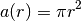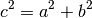Lecture 4 — Modules, Methods = Functions¶

• Material for this lecture is drawn from Chapter 4 of Practical Programming.
• We will first concentrate on program structure.
• We will then talk on using existing modules, including the ones you write.
• One important use of modules is testing. We will talk about how to develop test modules.
• We will revisit all these concepts in later lectures.

What have we learnt so far?¶

• Variables and expressions are good for one time operations, but functions allow us to reuse the same computation many times.
• A program is a sequence of operations written in a file.
• A number of operations in a program simply define functions.
• A number of operations use these functions.
• A good program separates these out very carefully.

Basic program structure¶

• Programmers have developed some conventions over time to make programs easy to read. We will use the same in this class.
• Here is an example.
"""
Computes the current in a given circuit and prints the result.

"""

def electric_current(voltage,resistance):
""" Returns the electric current given voltage and resistance.
Assumes that the units are compatible. """

current = float(voltage)/resistance
return current

## This is another comment
## Main code starts here. Let's define our variables first.
v = 220   # voltage in volts
r = 10    # resistance in ohms
print v, "volts", r, "ohms"
print "results in", electric_current(v,r), "amps of current"
• The program starts with a comment in quotes that describes the purpose of the program. The comment is not executed.
• This is a good place to put your pseudo code.
• Then, a number of functions will be defined that will be later used in the program. All functions should have a clear purpose.
• The function only uses variables that are in its arguments or are explicitly assigned a value within the function. For example, current above is a variable local to the function.
• Then, the actual code of the program starts after all functions. The code typically calls these functions to do its work and print some results.
• The code often defines some variables, like v and r above to compute what it needs to compute.
• It is a great idea to assign values to these variables all together, if possible, right after all the functions so that they are easy to locate.
• If you want, explain what the variables stand for.
• Do programs written in other formats work? Sure. But, they are hard to understand and debug. We will be very insistent that you use this format.
• This will also prepare you for more strict languages like C, C++.

What happens when you run a program?¶

• It goes through each statement from the very beginning to the end.
• If the statement is a function definition, then it is remembered.
• If the statement calls a function or executes an expression, it is evaluated.
• What happens if your program only has function definitions?
"""
Contains functions for converting temperature from fahrenheit to celcius,
and from celcius to fahrenheit.

"""

def to_celcius(fahrenheit):
""" Returns the celcius equivalent of a temperature in fahrenheit. """

return (5.0/9) * (fahrenheit-zero_fahrenheit)

def to_fahrenheit(celcius):
""" Returns the celcius equivalent of a temperature in fahrenheit. """

return (9.0/5) * celcius + zero_fahrenheit

zero_fahrenheit = 32
• This program does not do anything, but contains functions that can be used in other programs. This is a module!

What is a Module?¶

• A collection of Python variables, functions and objects, all stored in a file

• We’ll define the notion of an object soon, but strings, ints and floats are all (built-in) objects.
• Modules allow code to be shared across many different programs.

• Before we can use a module, we need to import it:

>>> import module_name

• Importing makes the functions in the module available for use.

• Python has many, many modules to accomplish many different tasks, ranging from manipulating images to accessing Twitter feeds.

The Math Module¶

• An important example is the math module:

>>> import math
>>> math.sqrt(5)
2.2360679774997898
>>> math.trunc(4.5)
4
>>> math.ceil(4.5)
5.0
>>> math.log(1024,2)
10.0
>>> math.pi
3.1415926535897931

• We can get an explanation of what functions and variables are provided in a module using the help function

>>> import math
>>> help(math)

• We will work through an number of examples of using the math module in class.

Exercise Set 1¶

• Open Wing IDE, and create a new file.

• Cut and paste the temperature conversion code into a file. Save it in a file called temperature.py.

• In the python shell, try the following commands:

>>> import temperature
>>> help(temperature)
>>> help(temperature.to_celcius)
>>> temperature.zero_fahrenheit
>>> temperature.to_celcius(-10)
>>> temperature.to_fahrenheit(10)

• What is the difference between the math module and our program for temperature conversion?

• We see that all our comments become a documentation for our modules.
• Top comments are for the whole module, and the first comment after function definition is the function documentation.
• All the functions in class notes are documented in the same way to make it easy for you to understand and reuse them.
• Your modules may also use other modules.
• Make sure you put functions that share a common purpose into a single file.

Different Ways of Importing¶

• We can import only a selection of functions and variables:

>>> from math import sqrt,pi
>>> pi
3.141592653589793
>>> sqrt(4)
2.0

• Or we can give a new name to the module within our program:

>>> import math as m
>>> m.pi
3.141592653589793
>>> m.sqrt(4)
2.0

• Both of these methods helps us distinguish between the function sqrt and the data pi defined in the math module from a function with the same name (if we had one) in our program.

• We can also do this (which is NOT recommended!):

>>> from math import *

Now, there is no name difference between the math module functions and ours. It is dangerous, better avoid it.

Built-In Modules¶

• Type

>>> help(__builtins__)

to see all of the modules Python has built-in. These do not need to be imported.

• We can get help on any number of these. We’ll start with int, float and, especially, str.

• These are our first (of many) Python “objects”

Objects and Methods¶

• All variables in Python are objects.

• Objects are abstractions:

• They have a specific organization and structure to the data they store.
• They have operations/functions — we call them methods — applied to access and manipulate this data. specific to them.
• We know two object types already: int and float. Let us try the following:

• You can see now all the oeprations allowed on integers. There are multiple ways to call them.

You can always find out about different types of objects this way. Try the same with a float.

Exercise Set 2¶

1. Write a Python module that computes the area of a circle. Your module should use the math module. Remember, the formula is2. What happens when we type

import math
math.pi = 3

and then use math.pi?

Testing¶

• Testing is the most important part of writing a program. Almost no complex program is bug free the first time.
• If you change your code, you might fix a bug in one place, but introduce a bug elsewhere.
• Best way to write programs is to divide it into small parts, functions, test them carefully and build on them.
• Testing is not a one-time process, it continues as you change your program.
• We will start with a simple method for testing in this lecture, but learn more sophisticated ways to do this in the future.

Testing means expectations¶

• You write functions to compute something specific. Given a certain input, it should return a specific output.
• Testing should involve coming up with a set of input/output cases that can tell whether your function works correctly.
• What are good test values?
• Common values: input values you expect to be used in your program and you know what the output should be
• Values that can possibly cause errors in your program: strange input values that can cause issues such as very high and very low values, sometimes called edge cases.
• Let us try do think of some good test cases for the following function:
def entropy(p1,p2):
x = float(p1)/(p1+p2)
y = -x * math.log(x) - (1-x) * math.log(1-x)
return y

Writing test code¶

• Instead of manually typing in each test case, you can write a simple program to test your functions.
• Write your functions into a module, like the temperate.py module.
• Now, write code that checks the functions in this module by printing the expected output and the output returned by the function side by side.
import temperature as tempt

print 'input: 32 F, expected output: 0 C, returned output:', tempt.to_celcius(32)
print 'input: 0 C, expected output: 32 F, returned output:', tempt.to_fahrenheit(0)
print 'input: 10 C, expected output: 10 C, returned output:', \
tempt.to_celcius(tempt.to_fahrenheit(10)), 'returned'
• Should we change these test cases?

• Note that:

• the backslash, \, is a way to tell the current statement is continuing in the next line.

• print() function prints the result of different

expressions. When you have multiple expressions seperated with a comma, print separates them with a space.

Exercise Set 3¶

1. Using the math module and the math.sqrt() function, write a module that computes the length of the hypotenuse, given the length of the other edges given by the theorem:2. List a number of good test cases for this function. Discuss in class how the function should work for these test cases.

Summary¶

• Follow the programming convention of a single comment explaining your program purpose, all your functions and then the main program.

• When a piece of code only has variable assignments and function definitions, it can be loaded as a module.

• Modules contain a combination of functions, variables, object definitions, and other code, all designed for use in other Python programs and modules

• After they are imported, the functions in a module can be executed by a call of the form:

module_name.function_name(arguments)

• Comment all your functions to explain their purpose. These can be viewed when you run:

>>> help(module_name.function_name)

• Write test code for your functions that prints expected output side by side by the actual output. We will improve this after we learn if statements.

• Python has many modules that make it very easy to do many complicated tasks. If you do not believe it, try typing:

>>> import antigravity